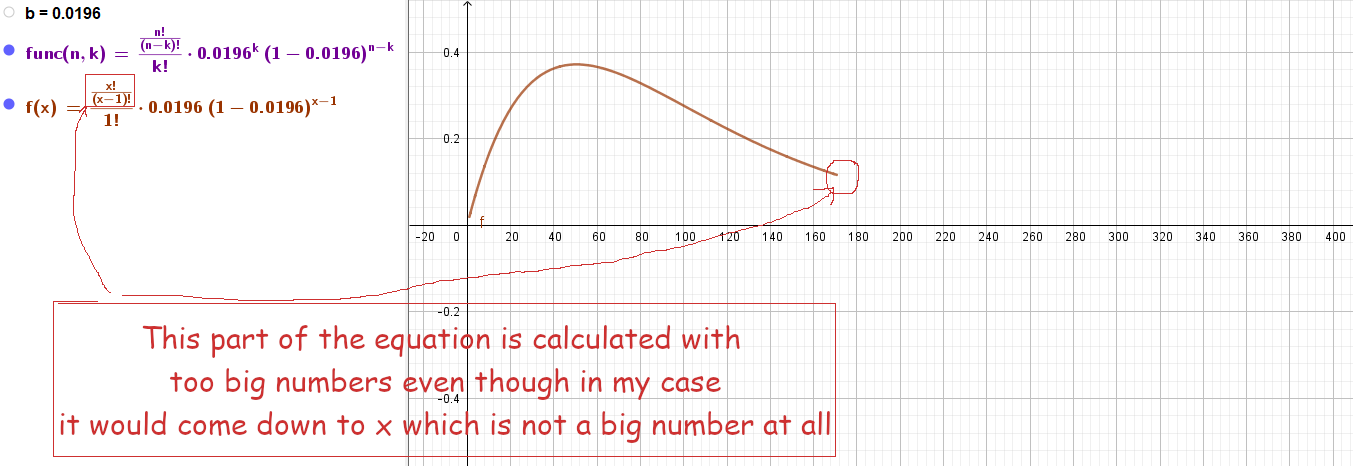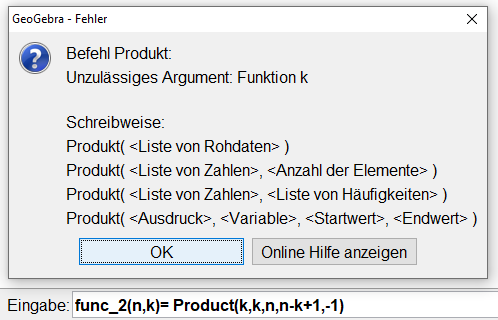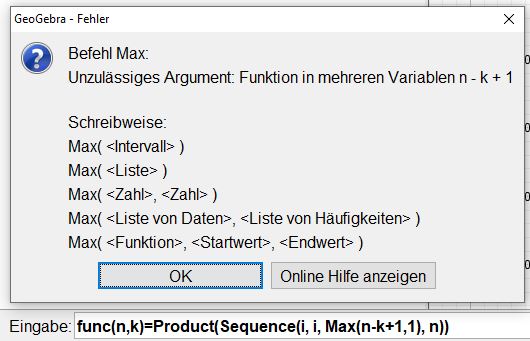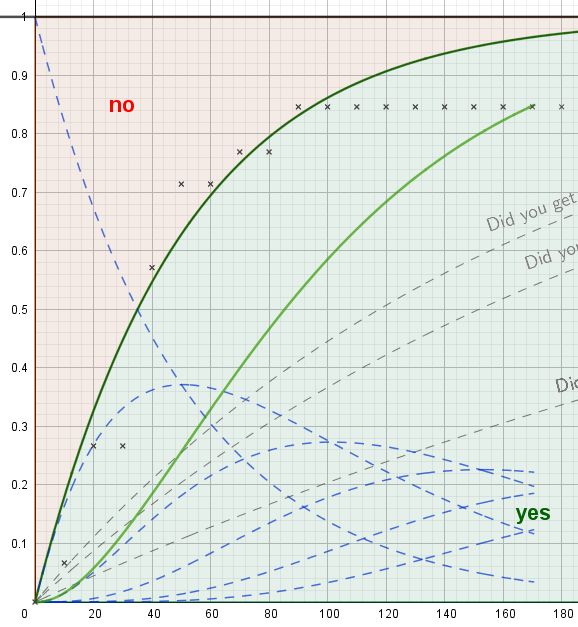# nCr results in too big numbers

doej1367 shared this question 2 years ago

Hi,

I have a problem with factorial producing too big number

`func(n, k) = n! / (n - k)! / k! b^k (1 - b)^(n - k)`

As n is the big number (around 0 to 500) and k the small one (interval 0 to 5) in my bernoilli experiment I would like to have a function that tells geogebra to (the following is Java-Code):

```myFunction(int n, int k) {
int product = 1;
for (int i = n; i > n-k; n--)
product *= i;
return product;
}```
to simplify this part of the equation and avoid the big numbers above '171!'
`n! / (n - k)!`

Is this possible with some javascript trickery or is it useless because GeoGebra is already smart enough to optimize it and this would not do anything at all?1

Please explain overall what you are trying to do1

Maybe with a picture then?this is why I would like to have a function that takes 2 parameters n and k and then multiplies n with (n-1) with (n-2) with (n-3) and so on... until (n-i) = (n-k+1) or (n-i) = 1

This would bring a (500! / (500 - 1)!) down to a 500

and a (500! / (500 - 2)!) down to 500*499

while 500! is basically impossible to calculate with a computer in java, 500*499 is very much possible and does not involve any extremely large numbers.

If you need context: this is just a formula that calculates the probability to succceed exactly k times in a bernoulli eperiment with p=0.0196 if you repeat it n times. n in func(n,k) is later defined as x to create a nice graph. k in func(n,k) is set to 0, 1, 2, 3, 4, 5, etc. to see the different graphs for exactly k successes in n tries.

Or in even simpler words: I want a function that takes 2 parameters to multiply all natural numbers from n to (n-k+1), with the possebility to set n to the variable x and k is just a fixed number. How do I do that?1

try Product(Sequence((n - k + 1) / k, k, 1, r))1

I did that ... there is just a minor problem with this - It doesn't let me do this:'GeoGebra Classic 5.0.620.0-d' in case that is important...1

And thats why I asked for some javascript trickery to add custom algorithms / functions.

It just doesn't like what i'm doing...

func(n,k)=Product(Sequence(i, i, Max(n-k+1,1), n))1

product and sequence are commads not functions

it is not possible to define functions using commands (ie: Max(f(x),g(x)) is not possible)

you can use a custom tool with Product(Sequence((n - k + 1) / k, k, 1, r))

Files: foro.ggb1

This almost works, sadly nPr gives 0 for non-integers

`nfunc(n,k) = nPr(n, k) / k! b^k (1 - b)^(n - k)`1

What do you want to do with it? Just plot graph, or calculate?1

as for right now i just want to plot a crap ton of graphs... from x = 0 to x = 500; but these graphs are all dependent on one another, meaning that I am adding / substracting graphs from one another to create new ones.

calculation of certain points and figuring out maxima etc. might come in later.1

Is there no way to tell geogebra to calc this n! / (n - k)! the smart way like a human would do it? Like a trim down first, calc later?2

Try like this using the CAS View and a trick...

Files: nPr3.ggb1

`b_k(x)=Ableitung(Integral(x! / (x - k)!)) / k! p^k (1 - p)^(x - k)`

nice trick with that integral and deduction. Not the most beautiful solution where I only need to put 2 numbers into a custom function - what I hoped for - but it works quite well.

Thx!1

We can probably make Polynomial((x!)/((x-2)!)) work in the CAS in the future (but mathmagic's solution below looks better :) )2

Veo que no entendí la petición correctamente cuando contesté con el anterior post en el que daba una herramienta que hacía pequeño el cálculo de nCr

viendo el truco de Michael no era tan complicado encontrar la llamada nPr2(x) porque basta con usar

g(x) = Polynomial(Product(Sequence(x - k, k, 0, n - 1))) que es lo mismo pero sin CAS y sin integrar

así b_k(x)=g(x)/k! p^k (1-p)^(x-k)